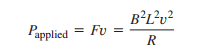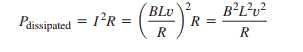# Energy Conservation between mechanical and electrical

• Physicslearner500039
U), then the potential difference (V) between A and B is given by V = U/q. This is a general formula and applies to circuits as well.In a circuit, the potential difference is usually created by a voltage source (such as a battery) which maintains a constant potential difference between its terminals. This potential difference can be used to move charges (current) through a resistor, for example, and the work done by the potential difference is dissipated as heat in the resistor.The distance (r) in the electrostatics formula is not applicable in circuits, as the potential difference is created along a conductor, not a fixed distance from a point charge. Instead, the distance can be thought of

#### Physicslearner500039

Homework Statement
The rate at which work is done is exactly equal to the rate at which energy is dissipated in the resistance.
Relevant Equations
NA
I am not sure if i can explain my question properly. I am studying the Generators section in the magnetism chapter. As i mentioned the statement "The rate at which work is done is exactly equal to the rate at which energy is dissipated in the resistance". When the term dissipated is used does it mean wasted? (Having resistance to an element is good or bad?)My understanding is when a mechanical force is applied and it is converted to current (electrical energy) using resistance, I can use this current for example glowing a bulb etc. A part of this energy is also lost as heat in the resistance, but how much of heat is lost, how to measure it? Please advise.

It is difficult to answer this question without knowing the details of the generator circuit.
But since you are studying the "basics" of electromechanical energy conversion, I think it is better if you forget about losses for now. Consider the resistance itself in your description to be a bulb.
The mechanical "power" (rate of change of energy) supplied to the rotating body (simply called rotor) is Torque×Angular speed and it is equal to the electrical power dissipated in the bulb.

•Physicslearner500039
Hi.

You said “… when a mechanical force is applied and it is converted to current (electrical energy) using resistance …”

We have to be careful of wording. Mechanical force is notconverted to current. Force and current are entirely different - you can’t convert one to the other. But you can use force in the process of producing current. And note, resistance is not used to produce current.

‘Dissipated’ in physics often means ‘converted to waste heat’. But it is sometimes used with shades of meaning, so you have to be prepared to interpret it in context.

For current flowing through a resistor, ‘dissipated’ simply means converted to heat. The heat might be very useful! E.g. heating a cold room or (in the case of a filament bulb) making the filament hot enough to produce visible light.

Suppose P_applied = 100W. That means a force (torque really) does 100J of work on the generator each second.

Then each second, all of the 100J must be converted to some other form(s) of energy. That’s the law of conservation of energy.

Consider two examples.

If the generator supplies a filament bulb of efficiency3% say, then we get 3W of light power and 97W of thermal power leaving the bulb. We would usually say P_dissipated = 100W in this contex, because the light is produced as ‘thermal’ (black-body) radiation and is considered part of the 'heat'. In this case we can say:
P_applied = P_dissipated

If the generator supplies a 70% efficient motor lifting some weights, we would say the motor’s output power is 70W. This is useful mechanical power and is not ‘dissipation’. 30W are dissipated (heating the motor due to the motor’s internal resistance and friction between moving parts). So P_dissipated = 30W in this example. We can say:
P_applied =P_useful + P_dissipated

In real circuts, the windings of the generator and the connecting wires have some resistance (r) so if you are doing accurate calculations you would incude the power dissipated in these (I²r).

Hope that helps.

Last edited:
•Lnewqban and Physicslearner500039
Steve4Physics said:
And note, resistance is not used to produce current.
I thought using Ohm's law ##V = I * R## and I am assuming that current is produced if we have voltage source ##V## and the circuit is having resistance ## R ##. So, probably there is a current source which can store current and does not require resistance to produce current. Am I correct?

Hi.

There are some misconceptions in what you wrote. I'm not sure I can clarify them all, but see if this helps.

If you have a voltage across zero resistance you (theoretically) get an infinite current. Resistance is not needed to produce the current – in fact the presence of resistance has opposite effect, it opposes a current flowing. (Infinite resistance will result in zero current.)

If you look at a thick connecting wire in a circuit, there can be a large current through the wire but the voltage between the ends of the wire and the wire's resistance are both almost zero.

You can’t store current under normal circumstance. (You need a superconducting circuit, the current flows round and round for ever - voltage and resistance are both zero in this case.)

(Terminology note. In electronics, a ‘current source’ is an electronic circuit/device which provides a constant current. To do this, it varies its output voltage automatically. It does not ‘store current’.)

Current is simply moving charge. You can store charge in a capacitor – while the charge enters or leaves you have a current.

A cell/battery does not store charge. Energy from chemical reactions drive electrons from one electrode to the other. The cell/battery acts as a pump – driving electrons already in the circuit's conductors - around the circuit.

You might find this video (explaining voltage and emf) helps:

•Lnewqban and Physicslearner500039
Thank you for clarifying and the videos are useful, but i have few doubts it will help if you can explain. In electric potential chapter i see the formula for electric potential for a point charge as ##V = \frac U q = \frac {q} {4\pi\epsilon 0 r}##. How to relate the above equation, in the electric circuit. What is ##r## here?

V = q/(4πε₀r) is a formula from the topic of electrostatics.

In the formula, V is the potential a distance r from a charge q (a point or spherically symmetric charge) in a vacuum. The formula is useful for some electrostatic calculations, but not relevant for circuit calculations.

However you should understand the general concept of voltage (potential difference) as it is the same for both electrostatics and for circuits.

Using your symbols, if you move a charge (q) from point A to point B and this requires an amount of energy (U) to be supplied, then the potential difference (V) between between A and B is:
V =U/q.

U can be thought of as the work done on the charge or the change in potential energy of the charge.

For example, suppose it takes 50J of energy to move a 10C charge from point A to point B. The voltage (potential difference) between A and B is:
V = U/q = 50J/10C = 5J/C
Since a 'volt' is just a name for 1J/C:
V = 5volts

A and B could be the ends of a resistor in a circuit, or 2 points in a vacuum. V = U/q applies on both cases. (But care is needed with + and – signs for the values of V, U and q.) I've oversimplified a bit in the interests of clarity/brevity.

•Physicslearner500039
Physicslearner500039 said:
Homework Statement:: The rate at which work is done is exactly equal to the rate at which energy is dissipated in the resistance.
Relevant Equations:: NA

"The rate at which work is done is exactly equal to the rate at which energy is dissipated in the resistance". When the term dissipated is used does it mean wasted? (Having resistance to an element is good or bad?)
I just baked an enchilada casserole in my oven. The heat generated in the resistor in this case was neither wasted or bad.

Value judgments are hard to make unless you know what the people you are talking to care about.

•Physicslearner500039